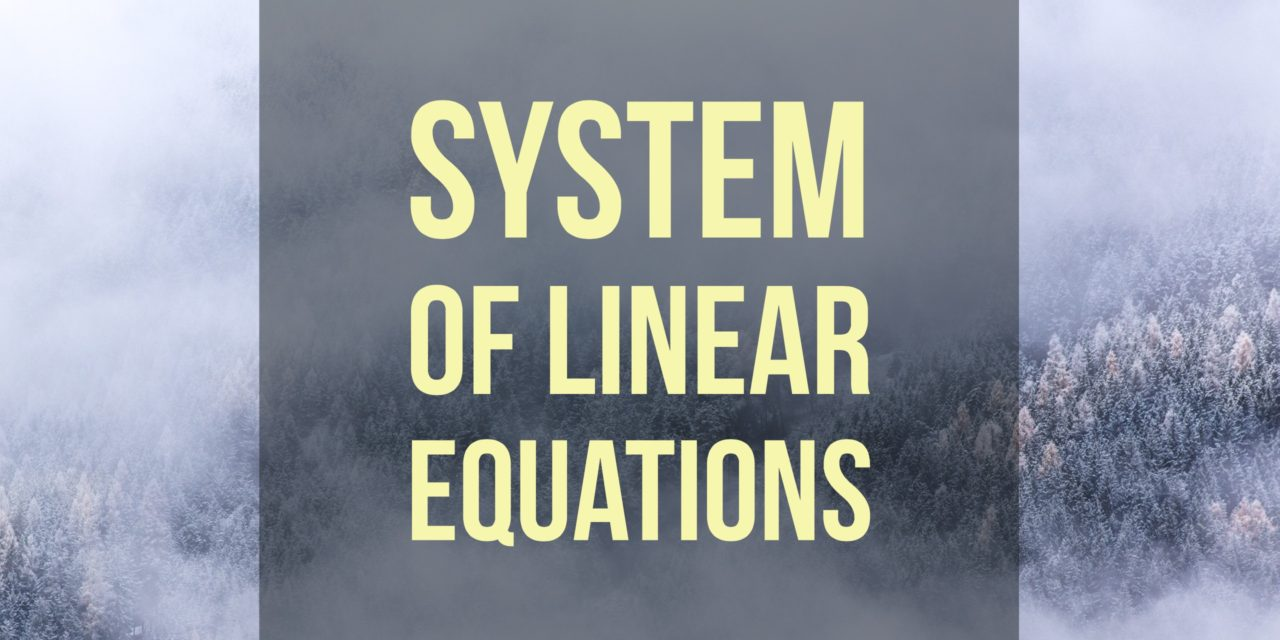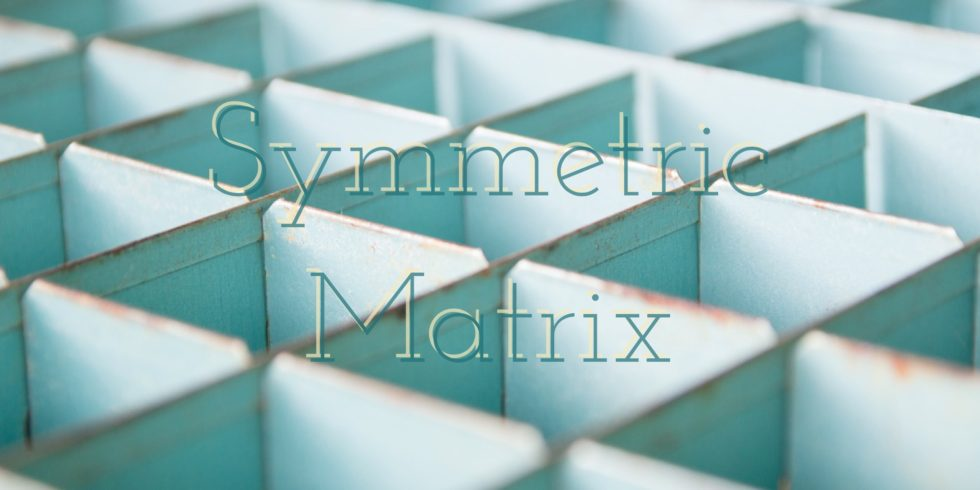# Determine Whether Matrices are in Reduced Row Echelon Form, and Find Solutions of Systems## Problem 648

Determine whether the following augmented matrices are in reduced row echelon form, and calculate the solution sets of their associated systems of linear equations.

(a) $\left[\begin{array}{rrr|r} 1 & 0 & 0 & 2 \\ 0 & 1 & 0 & -3 \\ 0 & 0 & 1 & 6 \end{array} \right]$.

(b) $\left[\begin{array}{rrr|r} 1 & 0 & 3 & -4 \\ 0 & 1 & 2 & 0 \end{array} \right]$.

(c) $\left[\begin{array}{rr|r} 1 & 2 & 0 \\ 1 & 1 & -1 \end{array} \right]$.Add to solve later

## Solution.

### (a) $\left[\begin{array}{rrr|r} 1 & 0 & 0 & 2 \\ 0 & 1 & 0 & -3 \\ 0 & 0 & 1 & 6 \end{array} \right]$

The first matrix is in reduced row echelon form.

For this matrix, we can read off the solution $x_1 = 2, x_2 = -3, x_3 = 6$.

### (b) $\left[\begin{array}{rrr|r} 1 & 0 & 3 & -4 \\ 0 & 1 & 2 & 0 \end{array} \right]$

The second matrix is in reduced row echelon form.

For this matrix, the variable $x_3$ is a free variable because there are no leading 1s in the 3rd column.

The solution set can be expressed as $x_1 = -4 – 3 x_3$, $x_2 = -2 x_3$, and $x_3$ can be any real number.

### (c) $\left[\begin{array}{rr|r} 1 & 2 & 0 \\ 1 & 1 & -1 \end{array} \right]$

The third matrix is not in reduced echelon form because the bottom-left entry is $1$, not $0$, so we first use elementary row operations to put it in this form.
\begin{align*}
\left[\begin{array}{rr|r} 1 & 2 & 0 \\ 1 & 1 & -1 \end{array} \right] \xrightarrow{R_2 – R_1} \left[\begin{array}{rr|r} 1 & 2 & 0 \\ 0 & -1 & -1 \end{array} \right]\\[6pt] \xrightarrow{(-1) R_2 } \left[\begin{array}{rr|r} 1 & 2 & 0 \\ 0 & 1 & 1 \end{array} \right] \xrightarrow{R_1 – 2 R_2} \left[\begin{array}{rr|r} 1 & 0 & -2 \\ 0 & 1 & 1 \end{array} \right].
\end{align*}
With the reduced matrix, we can read off the solution $x_1 = -2$ and $x_2 = 1$.Add to solve later

### More from my site

#### You may also like...

This site uses Akismet to reduce spam. Learn how your comment data is processed.

###### More in Linear Algebra##### If a Symmetric Matrix is in Reduced Row Echelon Form, then Is it Diagonal?

Recall that a matrix $A$ is symmetric if $A^\trans = A$, where $A^\trans$ is the transpose of $A$. Is it...

Close